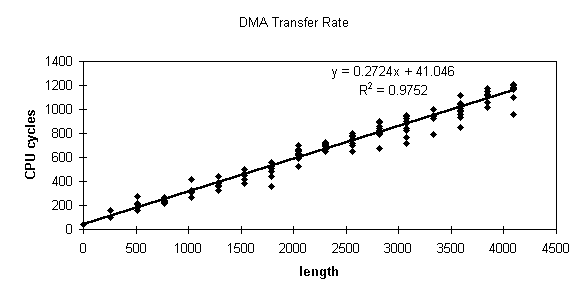This is the result of a test that I did of the speed of the N64's RSP DMA transfer. I got these results by starting a DMA with these different values in the DMA length register, then measuring the time until the DMA busy flag was turned off using the cop0 count register. This register increments at 1/2 CPU clock rate, so I doubled the value. As can be seen in the graph, the rate is about 0.27 cycles per byte, or 3.7 bytes per cycle. I don't have the code that I used for this test at my fingertips, but I'm sure that it didn't impact this experiment too severely. It was based around a loop that waited for the flag to be set. When length=0, the flag was set by the time it was first tested, so the base value for the transfer time, 41 cycles, is probably the loop overhead. Note that the value in length is 8 bytes less than the actual length transferred, so there is actually some work done when length=0.
The R2 value given, .9752, is a measure of the goodness of fit of the line to the data. The closer this value is to one, the more closely a straight line fits the data. (Since I took a statistics course I must say that this is not said entirely right, but it doesn't matter). An R2 of .9752 is close enough to conclude that there is a very high probability that there is a direct linear relationship between the number of bytes you want to transfer and the time it takes to do so.
Taking into account the fact that you can have one DMA queued while another is executing, and that you can still run both the CPU and the RSP parallel to the DMA, it is a very, very effective method of quickly moving data around.
Back to N64 Stuff